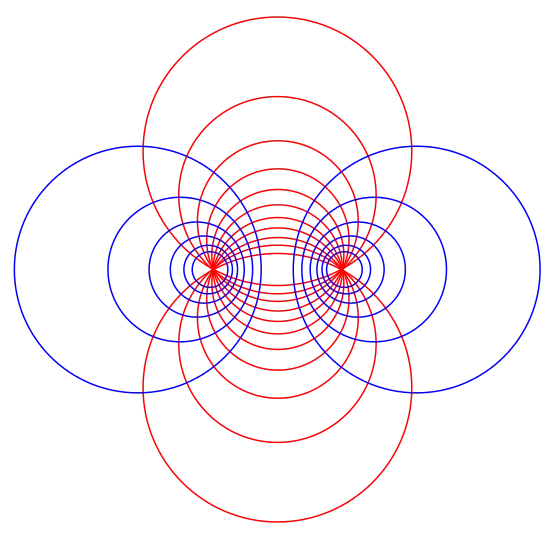# Tag differential equations all concepts

## Differential Equations Class 12 notes | IIT-JEE study materialSo, in calculus we are starting the topic of differential equations, we will be seeing what does a differential equation exactly means, what are partial differential equations, the significance of differential equations in various disciplines is very vast, we will…